# Pauli spin matrices

The Pauli spin matrices (named after physicist Wolfgang Ernst Pauli) are a set of unitary Hermitian matrices which form an orthogonal basis (along with the identity matrix) for the real Hilbert space of 2 × 2 Hermitian matrices and for the complex Hilbert spaces of all 2 × 2 matrices. They are usually denoted: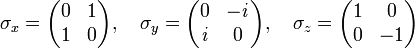$\sigma_x=\begin{pmatrix} 0 & 1 \\ 1 & 0 \end{pmatrix}, \quad \sigma_y=\begin{pmatrix} 0 & -\mathit{i} \\ \mathit{i} & 0 \end{pmatrix}, \quad \sigma_z=\begin{pmatrix} 1 & 0 \\ 0 & -1 \end{pmatrix}$

## Algebraic properties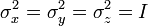$\sigma_x^2=\sigma_y^2=\sigma_z^2=I$

For i = 1, 2, 3: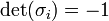$\mbox{det}(\sigma_i)=-1\,$$\mbox{Tr}(\sigma_i)=0\,$$\mbox{eigenvalues}=\pm 1\,$

### Commutation relations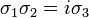$\sigma_1\sigma_2 = i\sigma_3\,\!$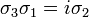$\sigma_3\sigma_1 = i\sigma_2\,\!$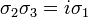$\sigma_2\sigma_3 = i\sigma_1\,\!$$\sigma_i\sigma_j = -\sigma_j\sigma_i\mbox{ for }i\ne j\,\!$

The Pauli matrices obey the following commutation and anticommutation relations:$\begin{matrix} [\sigma_i, \sigma_j] &=& 2 i\,\varepsilon_{i j k}\,\sigma_k \\[1ex] \{\sigma_i, \sigma_j\} &=& 2 \delta_{i j} \cdot I \end{matrix}$
where$\varepsilon_{ijk}$ is the Levi-Civita symbol, δij is the Kronecker delta, and I is the identity matrix.

The above two relations can be summarized as:$\sigma_i \sigma_j = \delta_{ij} \cdot I + i \varepsilon_{ijk} \sigma_k. \,$Some content on this page may previously have appeared on Citizendium.